# Search by Topic

#### Resources tagged with Addition & subtraction similar to Eight Dominoes:

Filter by: Content type:
Age range:
Challenge level:

### There are 211 results

Broad Topics > Calculations and Numerical Methods > Addition & subtraction### Eight Dominoes

##### Age 7 to 16 Challenge Level:

Using the 8 dominoes make a square where each of the columns and rows adds up to 8### Football Sum

##### Age 11 to 14 Challenge Level:

Find the values of the nine letters in the sum: FOOT + BALL = GAME### Score

##### Age 11 to 14 Challenge Level:

There are exactly 3 ways to add 4 odd numbers to get 10. Find all the ways of adding 8 odd numbers to get 20. To be sure of getting all the solutions you will need to be systematic. What about. . . .### Got it Article

##### Age 7 to 14

This article gives you a few ideas for understanding the Got It! game and how you might find a winning strategy.### Adding and Subtracting Positive and Negative Numbers

##### Age 11 to 14

How can we help students make sense of addition and subtraction of negative numbers?### Number Daisy

##### Age 11 to 14 Challenge Level:

Can you find six numbers to go in the Daisy from which you can make all the numbers from 1 to a number bigger than 25?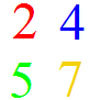### And So on and So On

##### Age 11 to 14 Challenge Level:

If you wrote all the possible four digit numbers made by using each of the digits 2, 4, 5, 7 once, what would they add up to?### Aba

##### Age 11 to 14 Challenge Level:

In the following sum the letters A, B, C, D, E and F stand for six distinct digits. Find all the ways of replacing the letters with digits so that the arithmetic is correct.### Digit Sum

##### Age 11 to 14 Challenge Level:

What is the sum of all the digits in all the integers from one to one million?### Escape from the Castle

##### Age 7 to 11 Challenge Level:

Skippy and Anna are locked in a room in a large castle. The key to that room, and all the other rooms, is a number. The numbers are locked away in a problem. Can you help them to get out?### Making Maths: Double-sided Magic Square

##### Age 7 to 14 Challenge Level:

Make your own double-sided magic square. But can you complete both sides once you've made the pieces?### Pairs

##### Age 11 to 14 Challenge Level:

Ann thought of 5 numbers and told Bob all the sums that could be made by adding the numbers in pairs. The list of sums is 6, 7, 8, 8, 9, 9, 10,10, 11, 12. Help Bob to find out which numbers Ann was. . . .### Twenty20

##### Age 7 to 16 Challenge Level:

Fancy a game of cricket? Here is a mathematical version you can play indoors without breaking any windows.### Pair Sums

##### Age 11 to 14 Challenge Level:

Five numbers added together in pairs produce: 0, 2, 4, 4, 6, 8, 9, 11, 13, 15 What are the five numbers?### More Children and Plants

##### Age 7 to 14 Challenge Level:

This challenge extends the Plants investigation so now four or more children are involved.### More Plant Spaces

##### Age 7 to 14 Challenge Level:

This challenging activity involves finding different ways to distribute fifteen items among four sets, when the sets must include three, four, five and six items.### Sort Them Out (2)

##### Age 7 to 11 Challenge Level:

Can you each work out the number on your card? What do you notice? How could you sort the cards?### Arrange the Digits

##### Age 11 to 14 Challenge Level:

Can you arrange the digits 1,2,3,4,5,6,7,8,9 into three 3-digit numbers such that their total is close to 1500?### The Patent Solution

##### Age 11 to 14 Challenge Level:

A combination mechanism for a safe comprises thirty-two tumblers numbered from one to thirty-two in such a way that the numbers in each wheel total 132... Could you open the safe?### Jugs of Wine

##### Age 11 to 14 Challenge Level:

You have four jugs of 9, 7, 4 and 2 litres capacity. The 9 litre jug is full of wine, the others are empty. Can you divide the wine into three equal quantities?### Weights

##### Age 11 to 14 Challenge Level:

Different combinations of the weights available allow you to make different totals. Which totals can you make?### Kids

##### Age 11 to 14 Challenge Level:

Find the numbers in this sum### Top-heavy Pyramids

##### Age 11 to 14 Challenge Level:

Use the numbers in the box below to make the base of a top-heavy pyramid whose top number is 200.### Chameleons

##### Age 11 to 14 Challenge Level:

Whenever two chameleons of different colours meet they change colour to the third colour. Describe the shortest sequence of meetings in which all the chameleons change to green if you start with 12. . . .### Alphabet Soup

##### Age 11 to 14 Challenge Level:

This challenge is to make up YOUR OWN alphanumeric. Each letter represents a digit and where the same letter appears more than once it must represent the same digit each time.### Like Powers

##### Age 11 to 14 Challenge Level:

Investigate $1^n + 19^n + 20^n + 51^n + 57^n + 80^n + 82^n$ and $2^n + 12^n + 31^n + 40^n + 69^n + 71^n + 85^n$ for different values of n.### As Easy as 1,2,3

##### Age 11 to 14 Challenge Level:

When I type a sequence of letters my calculator gives the product of all the numbers in the corresponding memories. What numbers should I store so that when I type 'ONE' it returns 1, and when I type. . . .### Pole Star Sudoku 2

##### Age 11 to 16 Challenge Level:

This Sudoku, based on differences. Using the one clue number can you find the solution?### Subtraction Surprise

##### Age 7 to 14 Challenge Level:

Try out some calculations. Are you surprised by the results?### Cayley

##### Age 11 to 14 Challenge Level:

The letters in the following addition sum represent the digits 1 ... 9. If A=3 and D=2, what number is represented by "CAYLEY"?### Making Sense of Positives and Negatives

##### Age 11 to 14

This article suggests some ways of making sense of calculations involving positive and negative numbers.### Consecutive Negative Numbers

##### Age 11 to 14 Challenge Level:

Do you notice anything about the solutions when you add and/or subtract consecutive negative numbers?### Odds and Threes

##### Age 7 to 11 Challenge Level:

A game for 2 people using a pack of cards Turn over 2 cards and try to make an odd number or a multiple of 3.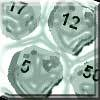### Asteroid Blast

##### Age 7 to 11 Challenge Level:

A game for 2 people. Use your skills of addition, subtraction, multiplication and division to blast the asteroids.### Zargon Glasses

##### Age 7 to 11 Challenge Level:

Zumf makes spectacles for the residents of the planet Zargon, who have either 3 eyes or 4 eyes. How many lenses will Zumf need to make all the different orders for 9 families?### Polo Square

##### Age 7 to 11 Challenge Level:

Arrange eight of the numbers between 1 and 9 in the Polo Square below so that each side adds to the same total.### One Million to Seven

##### Age 7 to 11 Challenge Level:

Start by putting one million (1 000 000) into the display of your calculator. Can you reduce this to 7 using just the 7 key and add, subtract, multiply, divide and equals as many times as you like?### Sheep Talk

##### Age 7 to 11 Challenge Level:

In sheep talk the only letters used are B and A. A sequence of words is formed by following certain rules. What do you notice when you count the letters in each word?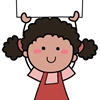### Carrying Cards

##### Age 7 to 11 Challenge Level:

These sixteen children are standing in four lines of four, one behind the other. They are each holding a card with a number on it. Can you work out the missing numbers?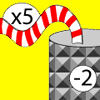### Function Machines

##### Age 7 to 11 Challenge Level:

If the numbers 5, 7 and 4 go into this function machine, what numbers will come out?### The Money Maze

##### Age 7 to 11 Challenge Level:

Go through the maze, collecting and losing your money as you go. Which route gives you the highest return? And the lowest?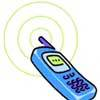### Mobile Numbers

##### Age 5 to 11 Challenge Level:

In this investigation, you are challenged to make mobile phone numbers which are easy to remember. What happens if you make a sequence adding 2 each time?### Number Differences

##### Age 7 to 11 Challenge Level:

Place the numbers from 1 to 9 in the squares below so that the difference between joined squares is odd. How many different ways can you do this?### Diagonal Sums

##### Age 7 to 11 Challenge Level:

In this 100 square, look at the green square which contains the numbers 2, 3, 12 and 13. What is the sum of the numbers that are diagonally opposite each other? What do you notice?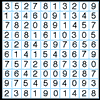### A Numbered Route

##### Age 7 to 11 Challenge Level:

Can you draw a continuous line through 16 numbers on this grid so that the total of the numbers you pass through is as high as possible?### Prompt Cards

##### Age 7 to 11 Challenge Level:

These two group activities use mathematical reasoning - one is numerical, one geometric.### Number Tracks

##### Age 7 to 11 Challenge Level:

Ben’s class were cutting up number tracks. First they cut them into twos and added up the numbers on each piece. What patterns could they see?### Napier's Bones

##### Age 7 to 11 Challenge Level:

The Scot, John Napier, invented these strips about 400 years ago to help calculate multiplication and division. Can you work out how to use Napier's bones to find the answer to these multiplications?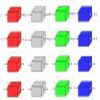### Machines

##### Age 7 to 11 Challenge Level:

What is happening at each box in these machines?### Pyramids

##### Age 11 to 14 Challenge Level:

What are the missing numbers in the pyramids?